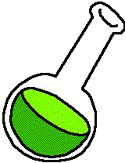#Acid-Base Chemistry By Kate Frueh

### Titrations:

Quantities of acid and base present in a sample, as well their pKa values can be determined from the analysis of titration curves. Three types of acid-base titrations are often used:

1) Titration of Strong Acid with Weak Base

2) Titration of Weak Acid and Strong Base

3) Tritration of Weak Base with Strong Acid

### Activity:

As bioanalytical chemists what we really care about is the activity of a substance. Activity is defined by Daniel C. Harris in Quantitative Chemical Analysis as "the value that replaces concentration in a thermodynamically correct equilibrium expression.

The activity of X is given by : Ax = [X]gc

Where gc is the activity coefficient and [X] is the concentration.

The activity coefficient is the number by which the concentration must be multiplied to get the activity.

The general form of the equilibrium constant is:

Using the above equation allows for the effect of ionic strength on the equilibrium because the activity depends on ionic strength.

Now you might be asking yourself, "How in the world am I ever going to find out what the activity coefficient, g, is?" Well, that's when we ask our good friends Debye and Huckel.

The Debye-Huckel Equation is:

log g = (-.51z2m1/2)/(1 + (am1.5/305))

Where g is the activity coefficient of an ion of charge +/-z and a is the size of the ion in pm in aqueous solution of ionic strength m.

Knowledge of a substance's activity is key to bioanalytical chemists and should always be used in place of a substance's concentration. So, if you ever catch yourself finding the pH of a solution using the [H+], take a step back and remember your g !

### Alpha Fractions:

Another very important part of acid-base chemistry for bioanalytical chemist is the notion of alpha fractions. For each species present in a titration, a fraction of it exists as either acid or base. This fraction will be helpful in several different titrations and equilibria.

In monoprotic systems-

The fraction of molecules in the form HA is:

aHA = [HA]/([HA] + [A-]) = [HA]/F = [H+]/([H+] + Ka)

Where F is the formal concentration and aHA is the fraction of the species in the form HA.

The fraction of molecules found as A- is:

aA- = [A-]/([A-] + [HA]) = [A-}/F = Ka/([H+] + Ka)

Where a is the fraction of HA that has dissociated.

The fraction association of a base is:

a = [BH+]/([BH+]+[B])

Where a for a base is equal to the fraction that has reacted with water.

Since much of bioanalytical chemistry involves diprotic acids as well as monprotic acids, a alpha fractions are integral to diprotic systems. The same pattern is used as was used above.

aH2A = H2A/F = [H+]/([H+]2 + [H+]K1 + K1K2)

Therefore,

aHA- = [HA-]/F = (K1[H+])/demoninator

and

aA2- = [A2-]/F = (K1K2)/denominator

Much of acid-base chemistry revolves around the use titration curves. However, in order to really understand and use the information, one must know how much of the species exists in each form. This is when our trusty alpha fractions come to the rescue. In order to carry out effective amino acid chemistry, alpha fractions are a necessity.

### References from Journal of Chemical Education:

Adams, K. R.; Meyers, M. B. Activation energies for an enzyme-catalyzed and acid catalyzed hydrolysis: An introductory interdisciplinary experiment for chemists and biochemists. J. Chem. Educ. 1985 62 86.

Bonham, Russell A. Determination of the Equilibrium Constants of a Weak Acid: An Experiment for Analytical or Physical Chemistry J. Chem. Educ. 1998 75 631. (May 1998)

Chirpich, Thomas P. "Rubberized" bone: A demonstration of solubility principles, acid base reactions, and biochemistry. J. Chem. Educ. 1986 63 519.

Hammett, Louis P. The theory of acids and bases in analytical chemistry. J. Chem. Educ. 1940 17 131.

Patterson, George S. A Simplified Method for Finding the pKa of an Acid-Base Indicator by Spectrophotometry J. Chem. Educ. 1999 76 395. (March 1999)

Rao, T. S.; Dabke, R. B.; Biradar, B. S. Equilibrium Constant of the Oxidation of Ascorbic Acid (Vitamin C): An Experiment in Biochemistry Laboratory. J. Chem. Educ. 1994 71 438.

Riyazuddin, P.; Devika, D. Potentiometric Acid-Base Titrations with Activated Graphite Electrodes J. Chem. Educ. 1997 74 1198. (October 1997)

Silva, Cesar R.; Simoni, Jose A.; Collins, Carol H.; Volpe, Pedro L. O. Ascorbic Acid as a Standard for Iodometric Titrations. An Analytical Experiment for General Chemistry J. Chem. Educ. 1999 76 1421. (October 1999)

Summerfield, John H. An Acid-Base Chemistry Example: Conversion of Nicotine J. Chem. Educ. 1999 76 1397. (October 1999)

Thompson, Robert Q. Identification of weak acids and bases by titration with primary standards: A modern version of an old analytical chemistry experiment. J. Chem. Educ. 1988 65 179.

### Some review articles that may be useful are:

Effects of lysine on ammonia formation, hydrogen ion, and potassium ion balance. Review and an hypothesis
Author: Zweig, Jack I. Source: Clin. Chem. 19, no. 9 (1973): 943-9

Review lecture. Central chemosensitivity and the reaction theory
Author: Loeschcke, H. H. Source: J. Physiol. (London) 332 (1982): 1-24, 1 plate

Strategies for the recovery of chemicals from fermentation: a review of the use of polymeric adsorbents
Author: Garcia, Antonio A. Source: Biotechnol. Prog. 7, no. 1 (1991): 33-42

### Some direction to link you to the acid/base chemistry world:

For interesting medical applications and interactive fun:

http://www.tmc.tulane.edu/departments/anesthesiology/acid/acid.html

For an acid-base tutorial and links to other acid/base web material:

http://astro.ocis.temple.edu/~piram/projects.html

For a quick review of acids, bases and pH:

http://web.jjay.cuny.edu/~acarpi/NSC/7-ph.htm

For a summary of acid-base chemistry:

http://hamers.chem.wisc.edu/chapman/AcidBase/summary.html